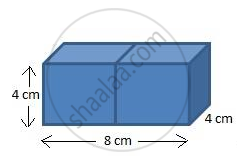Share

# Surface Area of a Combination of Solids

#### notes

We have already learnt about the surface area and volumes of figures, but in this chapter we will given different combinations of solids. We are known with solids like cylinder, sphere, cone, cuboid, rectangle, square, etc. In this chapter we are suppose to find surface areas of different combinations of solids.

Now before we proceed, we must understand what is curved surface area. The solids having curved surface are cone, cylinder. Curved surface area is the outer portion, the area of flat ends of such solids will not be included while calculating Curved surface area. But while calculating Total surface area we must include the area of flat ends of the solids, provided that the solid should not be hollow from inside.

Example- 2 cubes each of volume 64 cm^3 are joined end to end. Find the surface area of the resulting cuboid.

Solution- Volume   of   cube= 64cm^3

"edge"^3= 64cm^3

"edge of cube" = 3 sqrt 64 cm

edge= 4 cm

By adding this two cube we get a cuboidSo we get dimensions of cuboid as

As we have two cube the lenght will be doubled

therefore, lenght = 2(4)= 8cm

height= 4cm

Surface area of cuboid= 2 (lb+bh+hl)

= 2  (8 xx 4)+(4 xx 4)+(4 xx 8
)

= 2   (32+16+32)

= 2 (80)

Surface area of cuboid= 160 cm^2

#### Video Tutorials

We have provided more than 1 series of video tutorials for some topics to help you get a better understanding of the topic.

Series 1

Series 2

Series 3

### Shaalaa.com

Surface Area and Volume part 3 (Surface Area Examples) [00:11:20]
S
1
0%